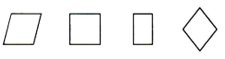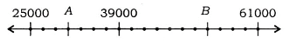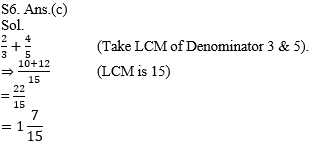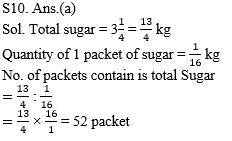### CTET/ UPTET Exam Practice Mathematics Questions

Dear Students!!! There is most general as well as a scoring section in all the competitive entrance examinations in the teaching field i.e "Mathematics".Because in this section only one thing is work i.e your accuracy and that could be nourished with the daily practice.With proper system, Study Notes, Quizzes, Vocabulary one can quiet his/her nerves and exceed expectations in the blink of an eye. So, for this, we are providing you the daily quiz for all teaching exams i.e CTET Exam 2019DSSSB ,KVS,STET Exam.

Q1.To develop skill of counting among children, which one of the following is not required to be learned as pre-number concept?

(a) One to one correspondence
(b) Seriation
(c) Reciting number names randomly
(d) Creating groups

(a) The child has no idea about the concept of area.
(b) The child used the area of rectangle as general idea of area of any closed shape.
(c) The child is right in saying area is length × breadth.
(d) The child is confused between the concept of area and peri-meter.

Q3. Which one of the following statements is true with respect to mathematics learning?

(a) Mathematics is a difficult subject to learn.
(b) Generally girls are weaker in mathematics.
(c) Everybody can learn mathematics.
(d) Mathematics can only be learnt by rigorous practice.

Q4. To teach the Pythagoras theorem, a teacher has distributed a sheet on which four right-angled triangles were drawn and asks the child to find the relationship between the sides of a triangle.
In the above situation, the teacher used—

(a) inductive method
(b) deductive method
(c) lecture method
(d) laboratory method

Q5. Which one of the following statements is not true about 'concept maps?

(a) Concept maps represent a collection of interconnected concepts and links connecting them.
(b) Concept maps should be / constructed by teachers only.
(c) Concept maps are hierarchical in nature.
(d) Concept maps help in linking prior knowledge to new instruction.Q7. For the given shapes, which one of the following statements is not correct?(a) All are parallelograms
(b) One of them is not a parallelogram.
(c) Two of them are rhombuses.
(d) Two of them are rectangles.

Q8. Which one of the following represents the number 'eleven thousand eleven hundred eleven'?

(a) 111111
(b) 12111
(c) 11000110011
(d) XIXIXI

Q9. The points A and B represent numbers on a number line as shown below :
25000 A 39000 B 61000The distance between the points A and B is-

(a) 29000 units
(b) 84000 units
(c) 22 units
(d) 22000 units(a) 52
(b) 48
(c) 12
(d) 64

Solutions

S1. Ans.(c)

S2. Ans.(b)

S3. Ans.(c)

S4. Ans.(a)

S5. Ans.(b)S7. Ans.(b)

S8. Ans.(b)
Sol. ⇒ 11,000 + 1100 + 11= 12111